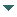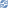# gvSIG

##### Personal tools
You are here: Home Operation invocation
gvSIG DesktopgvSIG Desktop

Cached time 11/21/13 11:24:39# Operation invocation

Up one level
 ◄ Previous Register operations Next: Project Site (documentación generada con maven) ►

This document explains how to invoke an operation associated to a geometry type

We only can invoke an operation if it had been registered previously. When it's registered, we can execute the operation in a geometry only with the operation's name. For example, to execute the PrintLn operation we will execute the next code (is assumed that exists a 2-dimensional point called point registered and the operation was registered for this geometry type).

```Object obj = point.invokeOperation("println", null);
```

The operations will return an object of the Object type with the result of the operation. In the invocation of the invokeOperation method appears a second parameter that is not used in the example. This argument is of the GeometryOperationContext type and it's a Map which contains all the input parameters for the operations.

In the next example we invoke an operation called intersects that is executed over two geometries. For that, is assumed that exists two Surface's in 2 dimensions called surface1 and surface2, previously created:

```GeometryOperationContext geomertyOperationContext = new GeometryOperationContext();
geometryOperationContext.setAttribute("geom2", surface2);
Boolean results = (Boolean)surface1.invokeOperation("intersects", geomertyOperationContext);
```

To make this code run, is supposed that the intersects operation is executed in a "context" where exists a second geometry which is registered with the name "geom2". This, increase the difficulty to use it, because it will necessary to know all the names of the objects that are used to create the correct context.

To avoid this, you can create a specific context classes to execute a concrete operation. In the intersects case, we can create a class like this:

```public class IntersectsGeometryOperationContext extends
GeometryOperationContext {

public IntersectsGeometryOperationContext(Geometry geom){
this.setGeom(geom);
}

public Geometry getGeom() {
return (Geometry)getAttribute("geom2");
}

public void setGeom(Geometry geom) {
setAttribute("geom2", geom);
}
}
```

Using this steps it isn't necessary to know the Map's name where the second geometry is stored. In the next example is going to execute the same code but this time is going to use the specific context for the operation:

```IntersectsGeometryOperationContext intersectsContext = new GeometryOperationContext(surface2);
Boolean results = (Boolean)surface1.invokeOperation("intersects", intersectsContext);
```

It isn't necessary to use the Map provided by the GeometryOperationContext. For that, you can create class attributes to store the context parameters and make a personal implementation.

Another possibility to execute operations is using their code. The operations have by agreement a static variable of the integer type which contains the operation code. To execute the associated code to the intersecs operation we will run the following code (is assumed that exists a IntersectsOperation class):

```IntersectsGeometryOperationContext intersectsContext = new GeometryOperationContext(surface2);
Boolean results = (Boolean)surface1.invokeOperation(Intersects.CODE, intersectsContext);
```

All this commented examples are used to execute an operation for a concrete geometry type. But it's interesting to bear in mind that we are passing the operation identificator by parameter to the geometry which will look for the operation to execute. In some cases, where there's a loop where are executed the same operation several times, the execution using this mechanism could be slow. For that, exists a way to do the operation access more efficiently.

The GeometryManager has a method called getGeometryOperation which returns an operation for a concrete geometry type and subtype. If, for example, we want to execute the intersects operation in a loop of 2-dimenasional Surface's (is assumed that we have all the surfaces in a variable of the type List<Surface> called surfaces) over a known surface (is also supposed that exists a variable of the type surface2) we can execute the next code:

```GeometryOperation intersectsOperation = geometryManager.getGeometryOperation(Intersects.CODE, Geometry.TYPES.Point, Geometry.SUBTYPES.GEOM2D);
IntersectsGeometryOperationContext intersectsContext = new GeometryOperationContext(surface2);
for (int i=0 ; i<surfaces.size() ; i++){
intersectsOperation.invoke(surfaces.get(i), intersectsContext);
}
```
 ◄ Previous Register operations Next: Project Site (documentación generada con maven) ►